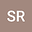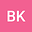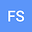Novel comparative analysis of fractional-order partial differential differential equations arising in quantum field theory pertaining to Caputo-Fabrizio derivative operator
•••• +1Bushra Kanwal
Fatima Jinnah Women University
Author ProfileFarhat SafdarIn this paper, we use the $\tilde{q}$-homotopy analysis transform method ($\tilde{q}$-HATM) to investigate the explicit solutions of the Kundu-Eckhaus equation and the massive Thirring model. Kundu-Eckhaus equation is a nonlinear partial differential equation found in quantum field theory, weakly nonlinear dispersive water waves, and nonlinear optics, as well as massive Thirring model, an exactly solvable quantum field theory that defines the self-interactions of a Dirac field in $(1+1)$ dimensions. The presented method combines the Yang transform methodology with the $\tilde{q}$-homotopy analysis technique and the fractional derivative described by the Caputo-Fabrizio (CF) operator in an intriguing way. The fixed point postulate is addressed in an attempt to illustrate that the resulting solution for the presented fractional order framework exists and is unique. We assess the predicted framework in the context of fractional order reinforcement and exhibit the performance of the prospective approach. The physical performance of the $\tilde{q}$-HATM solutions is also depicted using graphs for various fractional orders and simulation techniques.The present study demonstrated that the potential strategy is simple to apply and very systematic, productive, and precise in analyzing the behaviour of non-linear PDEs of fractional order that emerge in engineering and quantum field theory.